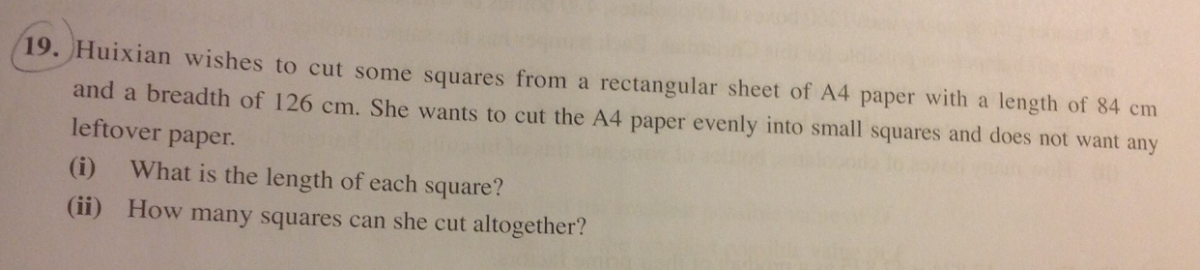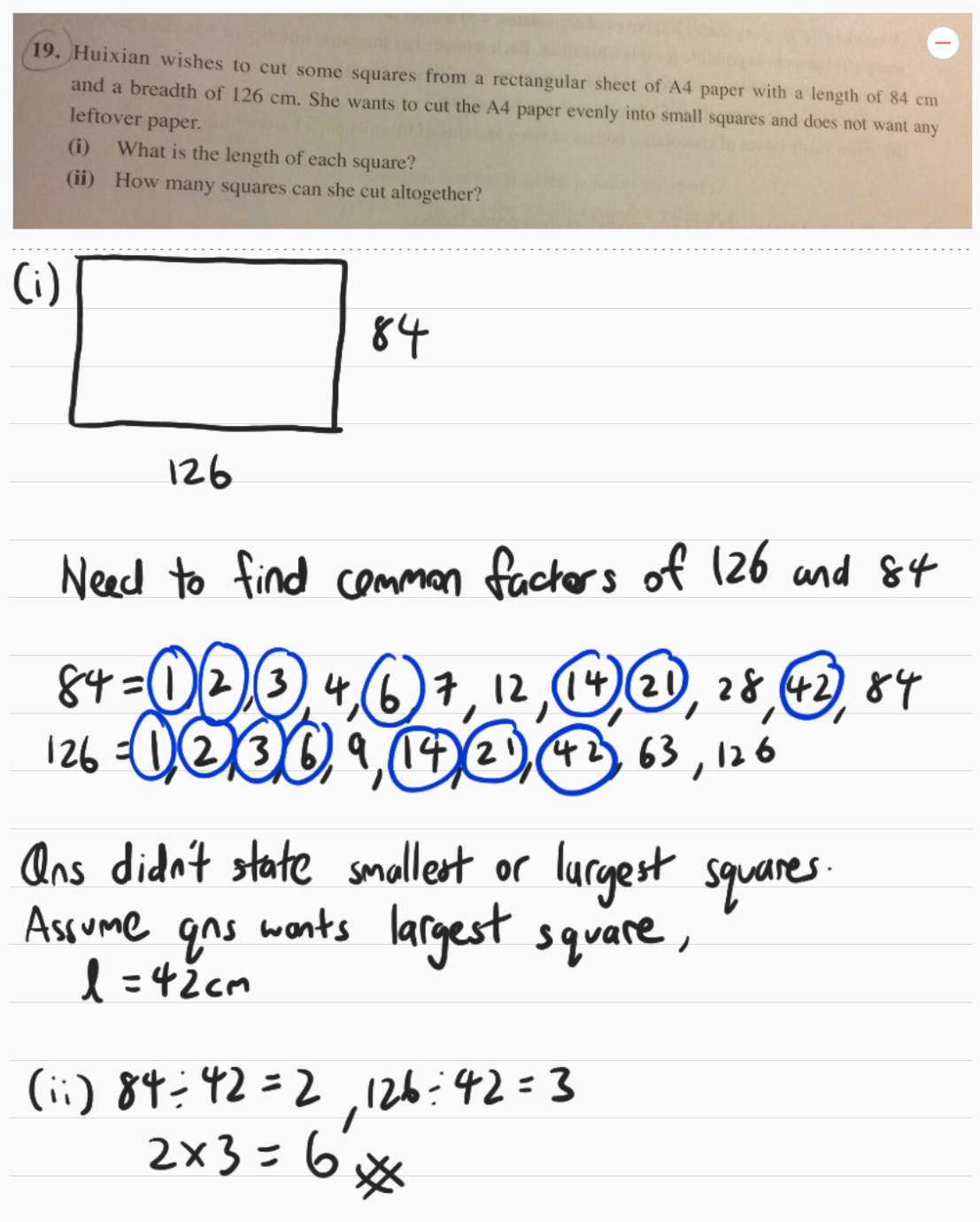QuestionHi,

Appreciate help on this. Thank you.

Source: Asses0 Replies 0 Likes

84 cm will be cut into a number of column of squares, so 84 divided the number of column of squares is the length of each square. This means the length of each square is a factor of 84

126 cm will be cut into a number of rows of squares, so 126 divided by the number of rows of squares is the length of each square. This means the length of each square is also a factor of 126

The length of each square is a common factor of 84 and 126

Common factors of 84 and 126 are 1, 2, 3, 6, 7, 14, 21, 42

(a) The length of each square can be any of the above numbers#

(b) The number of squares

When length is 1 cm: 84 x 126 ÷ 12 = 10584#

When length is 2 cm: 84 x 126 ÷ 22 = 2646#

When length is 3 cm: 84 x 126 ÷ 32 = 1176#

When length is 6 cm: 84 x 126 ÷ 62 = 294#

When length is 7 cm: 84 x 126 ÷ 72 = 216#

When length is 14 cm: 84 x 126 ÷ 142 = 54#

When length is 21 cm: 84 x 126 ÷ 212 = 24#

When length is 42 cm: 84 x 126 ÷ 422 = 6#

Hope it helps 🙂

0 Replies 0 Likes